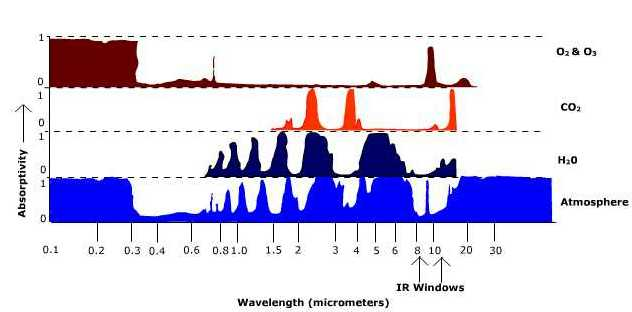# 4.1.3: Selective Absorption by the Atmosphere

$$\newcommand{\vecs}{\overset { \rightharpoonup} {\mathbf{#1}} }$$ $$\newcommand{\vecd}{\overset{-\!-\!\rightharpoonup}{\vphantom{a}\smash {#1}}}$$$$\newcommand{\id}{\mathrm{id}}$$ $$\newcommand{\Span}{\mathrm{span}}$$ $$\newcommand{\kernel}{\mathrm{null}\,}$$ $$\newcommand{\range}{\mathrm{range}\,}$$ $$\newcommand{\RealPart}{\mathrm{Re}}$$ $$\newcommand{\ImaginaryPart}{\mathrm{Im}}$$ $$\newcommand{\Argument}{\mathrm{Arg}}$$ $$\newcommand{\norm}{\| #1 \|}$$ $$\newcommand{\inner}{\langle #1, #2 \rangle}$$ $$\newcommand{\Span}{\mathrm{span}}$$ $$\newcommand{\id}{\mathrm{id}}$$ $$\newcommand{\Span}{\mathrm{span}}$$ $$\newcommand{\kernel}{\mathrm{null}\,}$$ $$\newcommand{\range}{\mathrm{range}\,}$$ $$\newcommand{\RealPart}{\mathrm{Re}}$$ $$\newcommand{\ImaginaryPart}{\mathrm{Im}}$$ $$\newcommand{\Argument}{\mathrm{Arg}}$$ $$\newcommand{\norm}{\| #1 \|}$$ $$\newcommand{\inner}{\langle #1, #2 \rangle}$$ $$\newcommand{\Span}{\mathrm{span}}$$$$\newcommand{\AA}{\unicode[.8,0]{x212B}}$$

The gasses that comprise our atmosphere are selective absorbers of radiation because each gas absorbs only particular wavelengths of light. Selective absorption by particular gasses and the atmosphere as a whole is shown in Figure $$\PageIndex{2}$$. The graph shows very little absorption for the atmosphere as a whole in the shortwave end of the spectrum, especially in the visible light band (the band of maximum emission for the Sun). The atmosphere absorbs far better in the longwave end of the electromagnetic spectrum which is the region of maximum emission (10$$\mu m$$) for the Earth.Figure $$\PageIndex{1}$$: Absorption of the atmosphere and selected gasses.

The IR windows are found at about 8 - 14 $$\mu m$$. It is through this portion of the electromagnetic spectrum that infrared radiation is transferred through the atmosphere and into outer space. These windows can be "closed" by clouds which absorb infrared radiation.

To understand why the atmosphere behaves as a selective absorber, see "Digging Deeper: Selective Absorption by the Atmosphere" or continue reading.

Digging Deeper into Radiation and Selective Absorption

So why is the atmosphere a selective absorber? The answer is found at the atomic level where electrons orbiting the nucleus of an atom are excited when struck by a bundle of energy. Though we describe electromagnetic radiation as invisible waves of energy, at the smallest scale it behaves as a particle, like when light is emitted by a single atom or molecule. When energy is given off, there is a change in the orbital pattern of the electrons that surround the nucleus of an atom. As the orbit changes, a bundle of energy called a "photon" is released. Particles of light differ from particles of matter: they have no mass, occupy no space, and travel at the speed of light, 2.9998 X 108 m s-1. The amount of energy carried by a photon varies inversely with wavelength, the shorter the wavelength, the more energetic the photon.

Electrons orbit the nucleus of an atom at fixed orbital distances called orbital shells. The orbital shell for each atom is different and discrete. That is, for a given atom like hydrogen, its electrons can only orbit at particular distances and are different than those for atoms of neon.Figure $$\PageIndex{2}$$: Effect of photon absorption on electron orbit.

Each orbital shell is associated with a given energy level; the greater the distance from the nucleus the greater the energy level. Electrons jump to a higher shell when excited by the absorption of energy. The photon must have the exact amount of energy to move the electron from, say, shell one to shell two. If the photon doesn't have enough energy to move the electron to shell two, it cannot move the electron half way between shell one and two. The atom does not stay in this excited, unstable state for very long. Energy is given off and the electron returns to a stable state or its "ground state" (lowest energy level or orbital distance). Recall that the amount of energy carried by a photon depends on the wavelength. Thus the atoms that comprise a gas can only absorb, or emit, particular wavelengths of energy (i.e. photons of energy).

This page titled 4.1.3: Selective Absorption by the Atmosphere is shared under a CC BY-SA 4.0 license and was authored, remixed, and/or curated by Michael E. Ritter (The Physical Environment) via source content that was edited to the style and standards of the LibreTexts platform; a detailed edit history is available upon request.